Importance: High ✭✭✭
 Author(s):
 Subject: Number Theory » Combinatorial Number Theory
 Keywords: Davenport constant subsequence sum zero sum
 Posted by: mdevos on: September 8th, 2007

For a finite (additive) abelian group, the Davenport constant of, denoted, is the smallest integerso that every sequence of elements ofwith length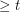has a nontrivial subsequence which sums to zero.

Conjecture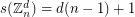Davenport's original motivation for introducing the constantconcerned prime ideal decompositions in algebraic number fields. However, determining this constant even for some very restricted families of groups has proved to be an interesting combinatorial problem. Indeed, the highlighted conjecture is considered to be one of the most important unsolved problems concerning finite abelian groups. I (M. DeVos) have reguarded this conjecture as folklore, but I await correction here.

It is easy to see that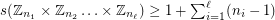because the sequence constructed by takingcopies of the element with ain the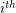position and's elsewhere has no nontrivial subsequence which sums to zero. There is also an easy upper bound of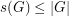. To see this, assume, let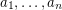be a sequence of elements from, and consider the terms. If these terms are distinct, then one must be 0 (giving us a zero sum subseqence). Otherwise two of them must be equal, so we havefor some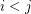, but then.

For cyclic groups, our trivial upper and lower bound match, so we have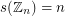. However, the situation gets much more difficult as soon as we go any further. The following theorem summarizes two classic results of Olson which remain state of the art.

Theorem  (Olson)
\item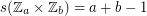if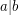. \item ifis prime,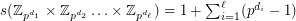Although there does not even exist a conjecture as to the value offor a general, recently a number of authors have proved theorems which give upper bounds onunder some structural assumptions. For instance, Caro has proved that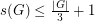for everywhich is not cyclic and not of the form.